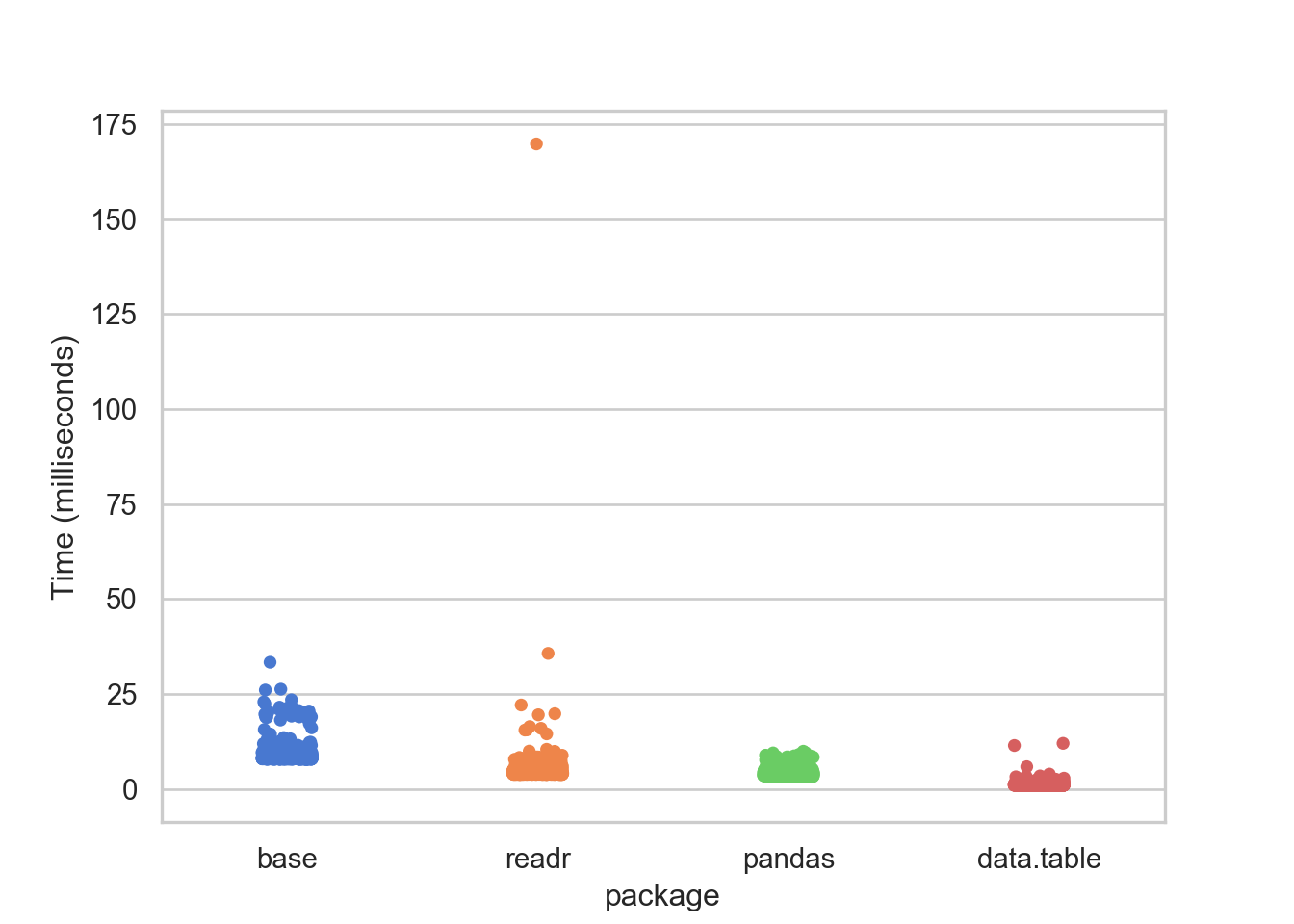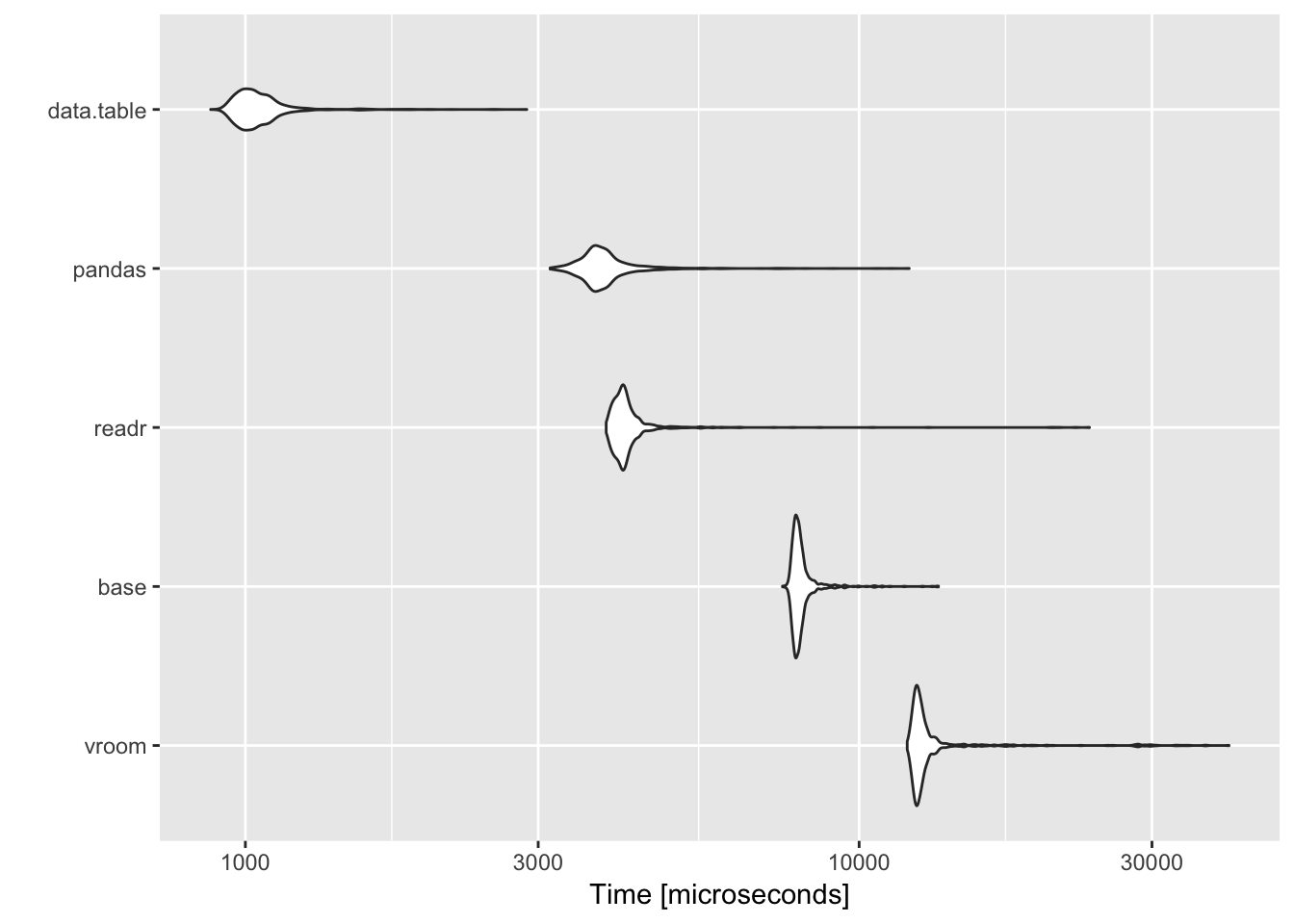What’s the fastest way to read a csv file in `R`? Among the `R` packages, the ultra-fast sprinter is certainly `data.table` but…few years ago, the introduction of the package `reticulate` gave us the possibility of use `python` and in particular the library `pandas` to read files in `R`. Would `pandas` (used in `R`) be faster than `data.table`? How would its performance compare with `readr` or base `R`? Let’s take a look.

# Benchmark evaluation

We start by loading few `R` libraries to read the csv file and evaluate the performance (`microbenchmark`) of our contestants…

 ``````1 2 3 4 `````` ``````library(tidyverse) library(reticulate) library(data.table) library(microbenchmark)``````

and by getting `pandas` in the `python` environment.

 ``````1 `````` ``import pandas as pd``

I downloaded the dataset that consists of 8437 observations of 4 variables and I placed on my home folder.

We are going to read the csv file using:

• base `R` function `read.csv`
• `pandas` function `read_csv`
• `readr::read_csv`
• `data.table::fread`

We read the file 1000 times for each of the package and record the performance with `microbenchmark::microbenchmark`

 `````` 1 2 3 4 5 6 7 8 9 10 11 12 13 `````` ``````mb <- microbenchmark( "base" = { read.csv("~/amis.csv", sep=",") }, "readr" = { read_csv("~/amis.csv") }, "pandas" = {py_run_string("pd.read_csv('~/amis.csv')") }, "data.table" = { fread("~/amis.csv") }, times = 1000)``````

And we summarize it

 ``````1 2 3 4 5 6 7 `````` ``````mb %>% group_by(expr) %>% rename(package = expr) %>% mutate(time_ms = time * 0.000001) %>% summarize(mean = mean(time_ms), median = median(time_ms), min = min(time_ms), max = max(time_ms), sd = sd(time_ms)) %>% arrange(mean) %>% knitr::kable(format = "html", caption = ": Milliseconds to read a csv file")``````
Table 1: : Milliseconds to read a csv file
package mean median min max sd
data.table 1.163429 1.051192 0.893294 11.988350 0.5746664
pandas 4.174706 3.900311 3.176870 9.903987 0.9339007
readr 4.763939 4.117349 3.787468 169.842847 5.5154385
base 8.979738 8.309741 7.746079 33.335507 2.4232146

The faster function is still `data.table::fread` with a mean reading time of about ~1 ms, followed by `pandas` (4.17 ms) and `readr` (4.76 ms). The R base function `read.csv` is the slowest, with reading times about 4-fold larger than `data.table::fread`.

We could graphically visualize the `microbenchmark` performance just launching `autoplot(mb)` but that would not be fun! We come so far, why not visualize the data using `python`?

# Seaborn

First we need to convert the `R` object `mb` to `python` object.

 ``````1 `````` ``py\$mb <- r_to_py(mb, convert = TRUE)``

In what is dataframe converted in `R`?

 ``````1 `````` ``type(mb)``
 ```1 ``` `## `

…of course in a `pandas Dataframe`.

Now, let’s import some libraries and plot the data using `seaborn`

 `````` 1 2 3 4 5 6 7 8 9 10 11 12 13 `````` ``````import numpy as np import seaborn as sns import matplotlib.pyplot as plt sns.set(style="whitegrid", palette="muted") mb['time_ms'] = mb['time'] * 0.000001 # from nano seconds to millisecond ax = sns.stripplot(x="expr", y="time_ms", data = mb) ax = ax.set(ylabel='Time (milliseconds)', xlabel='package') plt.show()``````# Conclusion

In this sprint race to import csv in `R`, the first place is still hold by the favourite `data.table::fread` followed by `pandas read_csv` and then by `readr::read_csv`. These two last packages/functions were really close at the final line. The base `R` function `read.csv` was not able to get to the podium and had reading times about 4-fold larger than `data.table::fread`.

Ciao Ciao!

# EDIT (05-08-2019): vroom!

Few days ago, vroom 1.0.0 was released on CRAN, and so we have another important contestant in our competition. Let’s look at an update table of the reading benchmark for the `amis.csv` file.

Table 2: : UPDATED Milliseconds to read a csv file
package mean median min max sd
data.table 1.060955 1.031286 0.877797 2.874855 0.1540645
pandas 3.897818 3.780800 3.137245 12.067028 0.6595005
readr 4.298844 4.128091 3.871632 23.730213 1.3351350
base 8.062673 7.951877 7.501073 13.467489 0.5136744
vroom 13.325987 12.528962 11.977720 40.054096 3.2400886

Under these conditions, `vroom` appears to be even slower than base `R` in terms of reading timesTo undestand why, we need to take a look at our `amis.csv` data.

 ```1 2 3 4 5 6 ``` ```## Classes 'data.table' and 'data.frame': 8437 obs. of 4 variables: ## \$ speed : int 26 26 26 26 27 28 28 28 28 29 ... ## \$ period : int 1 1 1 1 1 1 1 1 1 1 ... ## \$ warning: int 1 1 1 1 1 1 1 1 1 1 ... ## \$ pair : int 1 1 1 1 1 1 1 1 1 1 ... ## - attr(*, ".internal.selfref")=```

Our data consists of numeric variables and `vroom` advantage over the other packages/fucntions is that “character data is read from the file lazily; you only pay for the data you use”. So under these conditions, `data.table::fread` is still a gold medal!